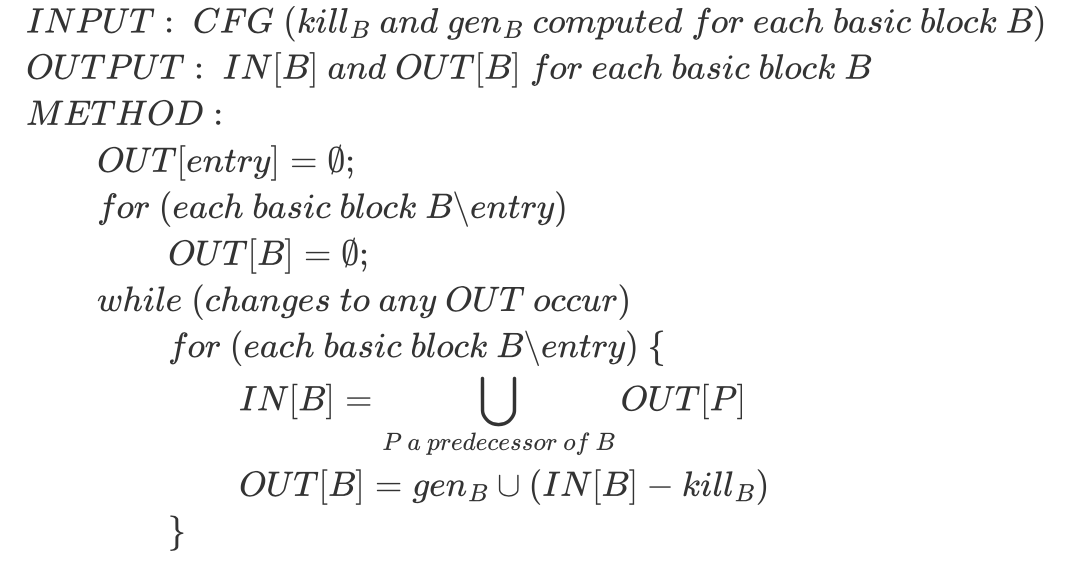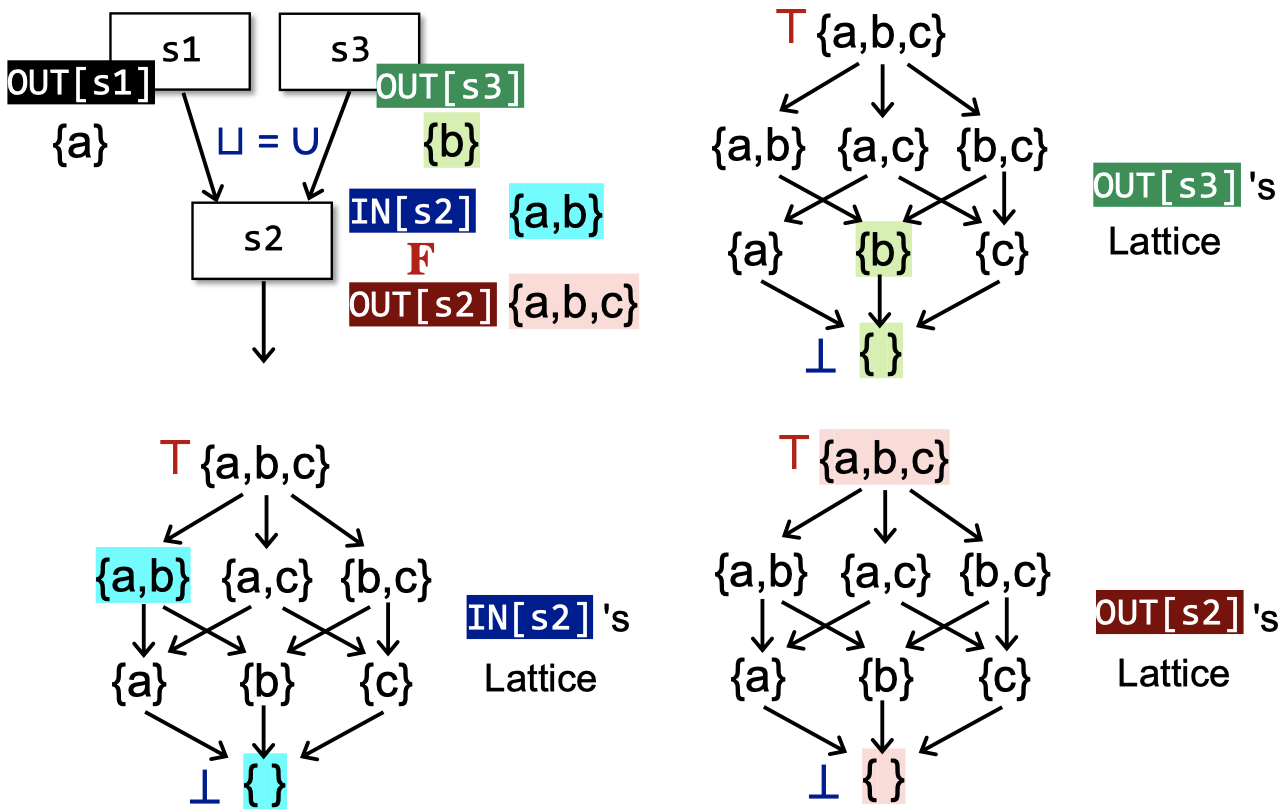## 再看迭代求解算法$$(OUT[n_{1}],\ OUT[n_{2}],\ …\ ,\ OUT[n_{k}])$$

$$iter\ i:\ (v_{1}^{i}, v_{2}^{i},\ …\ , v_{k}^{i}) = X_{i} = F(X_{i-1})$$

$$iter\ i+1:\ (v_{1}^{i+1}, v_{2}^{i+1},\ …\ , v_{k}^{i+1}) = X_{i+1} = F(X_{i})$$

$$X_{i} = X_{i+1} = F(X_{i})$$

1. 这个算法能够保证一定能终止（到达不动点/有解）吗？
2. 如果上一个问题的答案是肯定的，那么只有一个解/不动点吗？如果有多个不动点，哪一个是最好的、或者说最精确的？
3. 什么时候这个算法能够到达不动点呢？

## 偏序（Partial Order）

$$\forall\ x \in P, x \preccurlyeq x\ \ \text{(Reflexivity)}$$

$$\forall\ x,y \in P, x \preccurlyeq y \wedge y \preccurlyeq x \Longrightarrow x = y \ \ \text{(Antisymmetry)}$$

$$\forall\ x,y,z \in P, x \preccurlyeq y \wedge y \preccurlyeq z \Longrightarrow x \preccurlyeq z \ \ \text{(Transitivity)}$$

• 若$S$表示一个由整数组成的集合，$\preccurlyeq$表示“小于或等于”，那么$(S, \preccurlyeq)$就是一个poset。
• $S$表示一个由英文单词组成的集合，$\preccurlyeq$表示子串关系。
• $S$表示$\lbrace a, b, c \rbrace$的幂集（power set），$\preccurlyeq$表示子集关系。

graph TD A("{a, b, c}") --> B("{a, b}") A --> C("{a, c}") A --> D("{b, c}") B --> E("{a}") C --> E D --> E B --> F("{b}") C --> F D --> F B --> G("{c}") C --> G D --> G E --> H("{ }") F --> H G --> H

• 集合的包含关系是一种偏序。
• 在整数集中定义偏序：若a能整除b，我们就记为a≺b。

## 上界和下界（Upper/Lower Bounds）

graph TD A("{a, b, c}") --> B("{a, b}") A --> C("{a, c}") A --> D("{b, c}") B --> E("{a}") C --> E D --> E B --> F("{b}") C --> F D --> F B --> G("{c}") C --> G D --> G E --> H("{ }") F --> H G --> H style B fill:#11ee88 style C fill:#11ee88 style E fill:#11ee88 style F fill:#11ee88 style H fill:#11ee88

graph TD A("{a, b, c}") --> B("{a, b}") A --> C("{a, c}") A --> D("{b, c}") B --> E("{a}") C --> E D --> E B --> F("{b}") C --> F D --> F B --> G("{c}") C --> G D --> G E --> H("{ }") F --> H G --> H style B fill:#11ee88 style C fill:#11ee88 style E fill:#11ee88 style F fill:#11ee88

1. 不是所有的poset都有lub或glb。
2. 如果一个poset有lub或glb，它一定是唯一的。这一点可以借助偏序关系的antisymmetry特点证明。

## Lattice, Semilattice, Complete and Product Lattice

$$P = P_{1} \times\ …\ \times P_{n}$$

$$(x_{1},\ …\ , x_{n}) \preccurlyeq (y_{1},\ …\ , y_{n}) \Leftarrow \Rightarrow (x_{1} \preccurlyeq y_{1}) \wedge \ …\ \wedge (x_{n} \preccurlyeq y_{n})$$

$$(x_{1},\ …\ , x_{n})\ \lfloor \rfloor\ (y_{1},\ …\ , y_{n}) \Leftarrow \Rightarrow (x_{1} \lfloor \rfloor_{1} y_{1},\ …\ , x_{n} \lfloor \rfloor_{n} y_{n})$$

$$(x_{1},\ …\ , x_{n})\ \lceil \rceil\ (y_{1},\ …\ , y_{n}) \Leftarrow \Rightarrow (x_{1} \lceil \rceil_{1} y_{1},\ …\ , x_{n} \lceil \rceil_{n} y_{n})$$

## 基于格的数据流分析框架## 单调性（Monotonicity）和不动点（Fixed Point）定理

1. $f$的最小不动点可以通过以下方式得到：不断迭代计算$f(\bot), f(f(\bot)),\ …\ , f^{k}(\bot))$，直到到达一个不动点。
2. $f$的最大不动点可以通过类似的方式得到：不断迭代计算$f(\top), f(f(\top)),\ …\ , f^{k}(\top))$，直到到达一个不动点。

1. 证明不动点的存在

$$\bot \preccurlyeq f(\bot) \preccurlyeq f^{2}(\bot) \preccurlyeq\ …\ f^{H}(\bot)$$

2. 证明该不动点是最小不动点

1. 假设还有一个不动点$x$，使得$x = f(x)$。由于$\bot$是最小元素，结合函数单调性，可得$f(\bot) \preccurlyeq f(x)$。
2. 假设$f^{i}(\bot) \preccurlyeq f^{i}(x)$，那么结合函数单调性，我们有$f^{i+1}(\bot) \preccurlyeq f^{i+1}(x)$。
3. 因此，我们得证$f^{i}(\bot) \preccurlyeq f^{i}(x)$。又因为$x$是不动点，故$f^{i}(\bot) \preccurlyeq f^{i}(x) = x$。
4. 最终有$f^{Fix} = f^{k}(\bot) \preccurlyeq f^{k}(x) = x$，证毕。